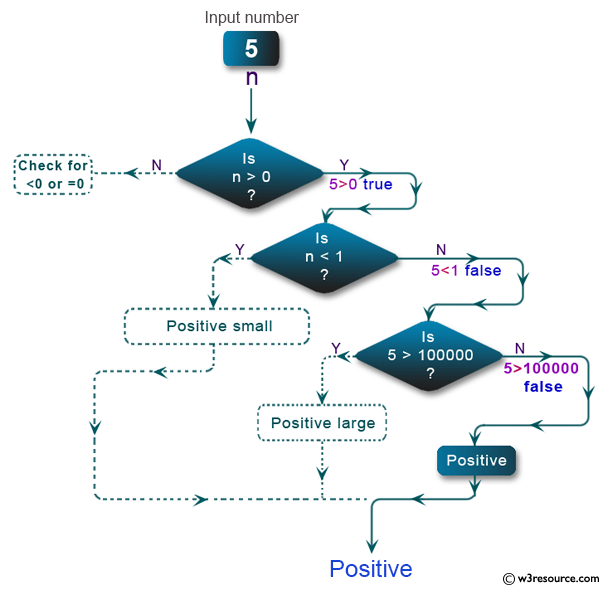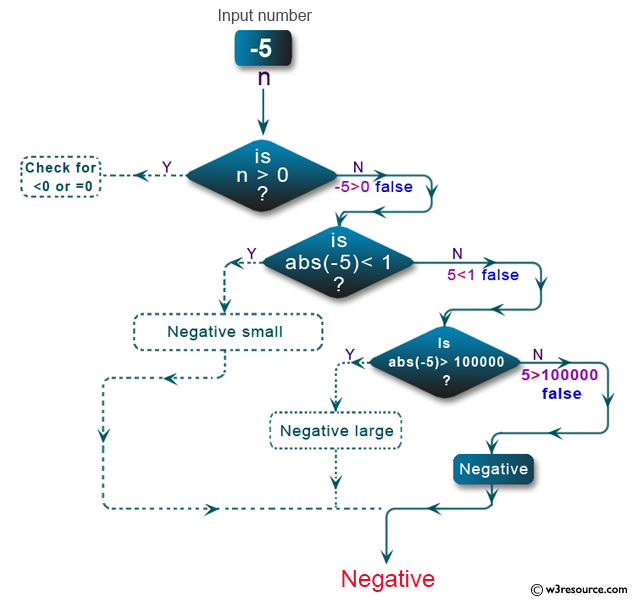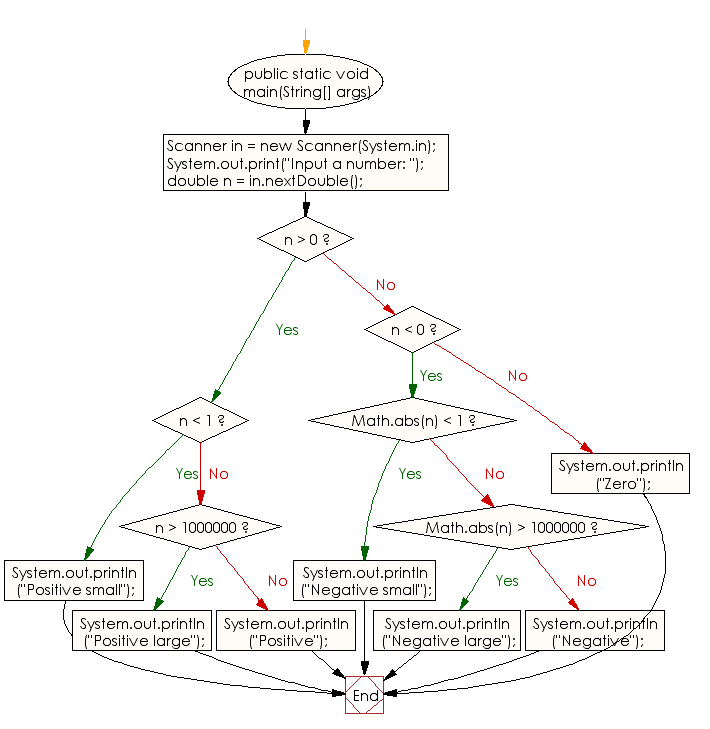﻿ Java exercises: Reads a floating-point number and print result - w3resource# Java Conditional Statement Exercises: Reads a floating-point number and print result

## Java Conditional Statement: Exercise-28 with Solution

Write a Java program that reads a floating-point number. If the number is zero it prints "zero", otherwise, print "positive" or "negative". Add "small" if the absolute value of the number is less than 1, or "large" if it exceeds 1,000,000.

Test Data
Input a number: -2534

Pictorial Presentation:Sample Solution:

Java Code:

``````import java.util.Scanner;
public class Exercise28 {

public static void main(String[] args)
{
Scanner in = new Scanner(System.in);
System.out.print("Input a number: ");
double n = in.nextDouble();

if (n > 0)
{
if (n < 1)
{
System.out.println("Positive small");
}
else if (n > 1000000)
{
System.out.println("Positive large");
}
else
{
System.out.println("Positive");
}
}
else if (n < 0)
{
if (Math.abs(n) < 1)
{
System.out.println("Negative small");
}
else if (Math.abs(n) > 1000000)
{
System.out.println("Negative large");
}
else
{
System.out.println("Negative");
}
}
else
{
System.out.println("Zero");
}
}
}
```
```

Sample Output:

```Input a number: -2534
Negative
```

Flowchart:Java Code Editor:

Improve this sample solution and post your code through Disqus

What is the difficulty level of this exercise?

﻿